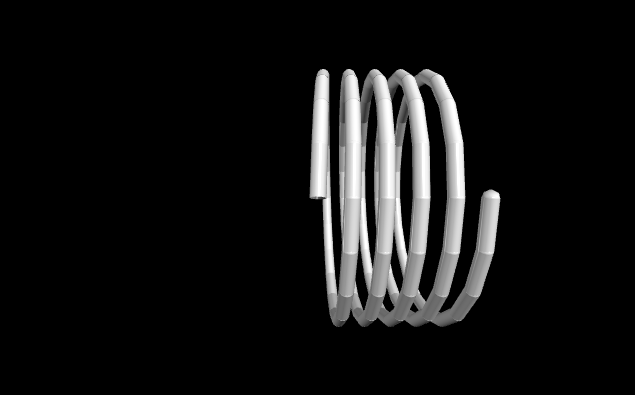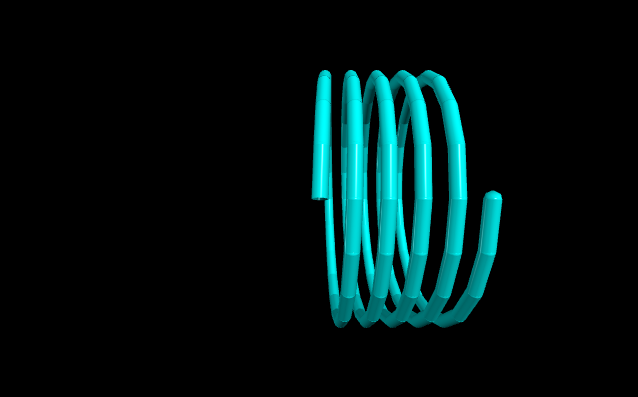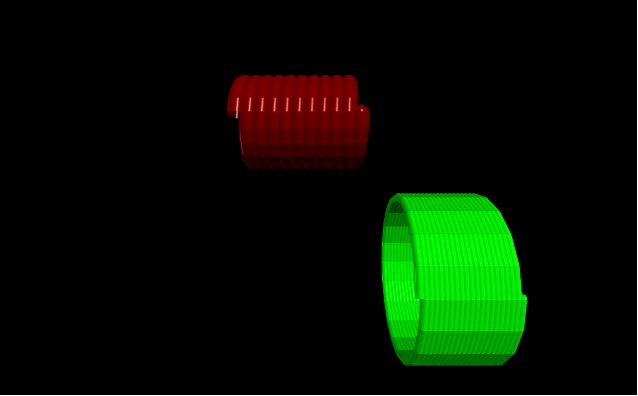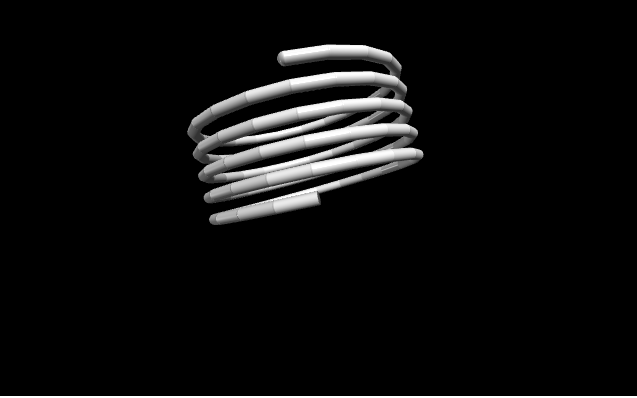# Making a helix with VPython

• Last Updated : 08 Jun, 2020

`VPython` makes it easy to create navigable 3D displays and animations, even for those with limited programming experience. Because it is based on Python, it also has much to offer for experienced programmers and researchers. `VPython` allows users to create objects such as spheres and cones in 3D space and displays these objects in a window. This makes it easy to create simple visualizations, allowing programmers to focus more on the computational aspect of their programs. The simplicity of `VPython` has made it a tool for the illustration of simple physics, especially in the educational environment.

Installation :

Attention geek! Strengthen your foundations with the Python Programming Foundation Course and learn the basics.

To begin with, your interview preparations Enhance your Data Structures concepts with the Python DS Course. And to begin with your Machine Learning Journey, join the Machine Learning - Basic Level Course

`pip install vpython`

A helix is a geometrical object in three-dimensional space which is shaped like a corkscrew or spiral staircase. It is a type of smooth space curve with tangent lines at a constant angle to a fixed axis. We can generate a helix in `VPython` using the `helix()` method.

## helix()

Syntax : helix(parameters)

Parameters :

• pos : It is the position of the center of one end of the helix. Assign a vector containing 3 values, example pos = vector(0, 0, 0)
• axis : It is the axis of alignment of the helix. Assign a vector containing 3 values, example axis = vector(1, 2, 1)
• color : It is the color of the helix. Assign a vector containing 3 values, example color = vector(1, 1, 1) will give the color white
• opacity : It is the opacity of the helix. Assign a floating value in which 1 is the most opaque and 0 the least opaque, example opacity = 0.5
• shininess : It is the shininess of the helix. Assign a floating value in which 1 is the most shiny and 0 the least shiny, example shininess = 0.6
• emissive : It is the emissivity of the helix. Assign a boolean value in which True is emissive and False is not emissive, example emissivity = False
• length : It is the length of the texture = textures.stucco. Assign a floating value, the default length is 1, example length = 10
• radius : It is the radius of the helix. Assign a floating value, the default radius is 1, example radius = 5
• coils : It is the number of coils in the helix. Assign an integer value, the default coils are 5, example coils = 9
• thickness : It is the diameter of the cross section of the curve used to draw the helix. Assign an floating value, the default thickness is radius/20, example thickness = 1
• size : It is the size of the helix. Assign a vector containing 3 values representing the length, height and width respectively, example size = vector(1, 1, 1)

All the parameters are optional.

Example 1 :A helix with no parameters, all the parameters will have the default value.

 `# import the module``from` `vpython ``import` `*` `helix()`

Output :Example 2 :A helix using the parameters color, opacity, shininess and emissivity.

 `# import the module``from` `vpython ``import` `*` `cone(color ``=` `vector(``0``, ``1``, ``1``), ``     ``opacity ``=` `0.5``, ``     ``shininess ``=` `1``, ``     ``emissive ``=` `False``)`

Output :Example 3 :Displaying 2 helices to visualize the attributes pos, length, radius, coils and thickness.

 `# import the module``from` `vpython ``import` `*`` ` `# the first helix``helix(pos ``=` `vector(``-``2``, ``2``, ``0``),``      ``length ``=` `3``,``      ``radius ``=` `1``,``      ``coils ``=` `10``,``      ``thickness ``=` `0.5``,``      ``color ``=` `vector(``0.5``, ``0``, ``0``))``  ` `# the second helix``helix(pos ``=` `vector(``1``, ``-``1``, ``5``), ``      ``color ``=` `vector(``0``, ``1``, ``0``),``      ``coils ``=` `20``)`

Output :Example 4 :A helix using the parameter axis and size.

 `# import the module``from` `vpython ``import` `*` `helix(axis ``=` `vector(``-``1``, ``4``, ``0``),``      ``size ``=` `vector(``1``, ``2``, ``2``))`

Output :My Personal Notes arrow_drop_up